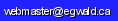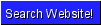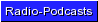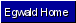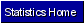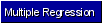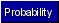Egwald Statistics: Probability and Stochastic Processes by Elmer G. Wiens Egwald's popular web pages are provided without cost to users. Please show your support by joining Egwald Web Services as a Facebook Fan:Follow Elmer Wiens on Twitter:Hypotheses Testing A. Testing the mean 1. Small sample from a normal population with known variance ø^2 Suppose you know that a random variable X has a normal distribution, but that you only know its variance ø^2. The sample mean of n observations, {X1, ...Xn} is Y = (X1 + ... + Xn)/n To test that the r.v. X has mean µ, use the fact that Z = (Y - µ)* sqrt(n) / ø has the standard normal distribution. If the absolute value of z is greater than 1.96, we can reject the hypotheses, at a 95% level of confidence, that the mean of X, E(X), is equal to µ.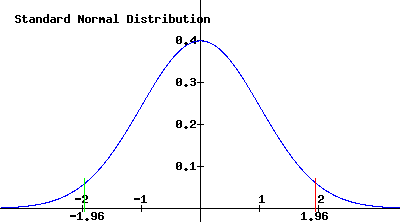2. Large sample, n >= 30, with unknown mean and (finite) variance The Central Limit Theorem permits us to approximate ø^2 with S^2 and assume that the r.v. Z = (Y - µ) * sqrt(n) / S has a standard normal distribution. 3. Small sample from a normal population whose mean and (finite) variance are unknown. If the sample mean = Y, and the sample variance = S^2 then the r.v. Z = (Y - µ) * sqrt(n) / S has a t-distribution with n-1 degrees of freedom. For example, suppose a sample of 10 observations yields a sample mean of y = 22 and a sample variance of s^2 = 7. We want to test the hypotheses that the population mean µ = 20. z = (22 - 20)* sqrt(10) / sqrt(7) = 2.39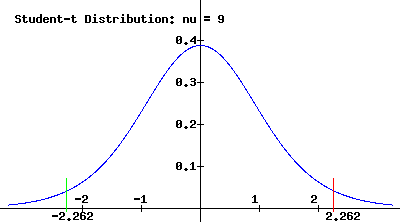Since 2.39 > 2.262 we can reject the hypotheses at the 95% confidence level. B. Testing the difference between means 1. Two independent random samples from two normal populations with known variances Let {X1, ... , Xn} be a sample of size n from the r.v. X with variance ø^2. Let {W1, ... , Wm} be a sample of size m from the r.v. W with variance ö^2. Compute their means as: Y = [X1+ ... + Xn] / n Z = [W1+ ... + Wm] / m Suppose we want to test the hypotheses: H0: y - z = þ against H1: y - z != þ The r.v. V defined by: V = [Y - Z - þ] / sqrt[(ø^2/n) + (ö^2/m)] has a standard normal distribution. We can reject the hypotheses if, for two samples, the value of V is larger than 1.96, or less than -1.96. 2. Two large, independent random samples (n,m >= 30) from two populations (not necessarily normal) with unknown variances The Central Limit Theorem permits us to approximate ø^2 with the sample variance S^2, and ö^2 with the sample variance R^2. Then V = [Y - Z - þ] / sqrt[(S^2/n) + (R^2/m)] has a standard normal distribution. For example: if for sample 1, y = 195, n = 35, s^2 = 45, and for sample 2, z = 200, m = 40, r^2 = 55. Suppose we want to test that the two populations have the same mean. Then v = [195 - 200 - 0] / sqrt[(45/35) + (55/40)] = -5 / 1.63 = -3.06 Using the standard normal distribution, we can reject the hypotheses, H0: y - z = 0, at the 95% confidence level. 3. Two small, independent random samples from two normal populations with the same variance ø^2 Suppose for sample 1, the sample mean = Y, the sample variance = S^2, and the sample size = n; for sample 2, the sample mean = Z, the sample variance = R^2, and the sample size = m. Then, the distribution of the r.v. T = [Y - Z - þ] / {sqrt[(n - 1)*S^2 + (m - 1)*R^2] * sqrt[1 / (n + m - 2)] * sqrt[(1/n) + 1/m)]} is the Student-t distribution with (n + m - 2) degrees of freedom.Copyright © Elmer G. Wiens:   Egwald Web ServicesAll Rights Reserved.    Inquiries